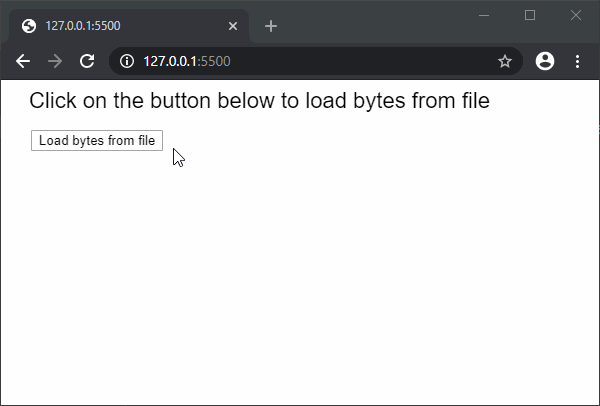• Last Updated : 27 Mar, 2020

The loadBytes() function is used to read the contents of a file or URL and return it as an object containing the series of bytes. The bytes can then be accessed by using the “bytes” property of the object. The file must be present in the sketch directory to be accessed. This method can support file sizes up to 64MB.

This function is asynchronous, therefore it is recommended to be called in the preload() function to ensure that the function is executed before the other functions.

Syntax:

`loadBytes(file, [callback], [errorCallback])`

Parameters: This function accept three parameters as mentioned above and described below:

• file: It is a string that denotes the file path or URL from where the XML data has to be loaded.
• callback: It is a function that is called when this function executes successfully. The first argument for this function is the XML data loaded from the file. It is an optional parameter.
• errorCallback: It is a function that is called if there is any error in executing the function. The first argument for this function is the error response. It is an optional parameter.

Return Value: It returns an object which has the “bytes” property set to the bytes loaded from the file.

Below examples illustrate the loadBytes() function in p5.js:

Example:

 `let loadedBytes = ``null``;``  ` `function` `setup() {``  ``createCanvas(500, 300);``  ``textSize(22);``  ` `  ``text(``"Click on the button below to "``    ``+ ``"load bytes from file"``, 20, 20);``  ` `  ``// Create a button for loading the XML``  ``loadBtn = createButton(``"Load bytes from file"``);``  ``loadBtn.position(30, 50)``  ``loadBtn.mousePressed(loadFileBytes);``}``  ` `function` `loadFileBytes() {``     ` `  ``// Load bytes from file``  ``loadedBytes = loadBytes(``'characters.txt'``, onFileload);``}``  ` `function` `onFileload() {``  ``text(``"Bytes loaded successfully..."``, 30, 100);``  ` `  ``// Print the bytes``  ``for` `(let i = 0; i < loadedBytes.bytes.length; i++)``    ``text(loadedBytes.bytes[i], 30 + i * 50, 150);``}`

Output:My Personal Notes arrow_drop_up